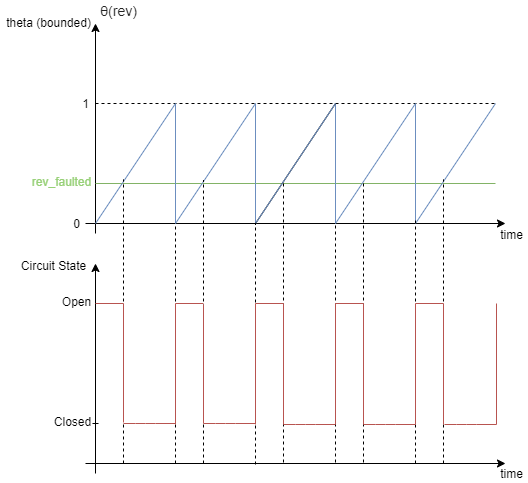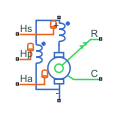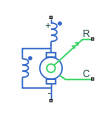# Compound Motor

Compound motor model with electrical and torque characteristics and fault modeling

Since R2021a

•Libraries:
Simscape / Electrical / Electromechanical / Brushed Motors

## Description

The Compound Motor block represents the electrical and torque characteristics of a compound motor. This figure shows the equivalent circuit for a short-shunt compound motor:This figure shows the equivalent circuit for a long-shunt compound motor:where:

• i is the total current.

• is is the series field winding current.

• ip is the parallel field winding current.

• ia is the armature current.

• V is the total voltage.

• Vs is the series field winding voltage.

• Vp is the parallel field winding voltage.

• Va is the armature voltage.

• ω is the angular velocity.

• te is the torque.

If you set the Steady-state parameterization parameter to `By equivalent circuit parameters`, you can specify the equivalent circuit parameters for this model:

• RaArmature resistance, Ra

• RsSeries field winding resistance, Rs

• RpShunt field winding resistance, Rp

• LsaSeries field winding to armature back EMF constant, Lsa

• LpaShunt field winding to armature back EMF constant, Lpa

### Short-Shunt Equations

When Electrical circuit topology is set to `Short-shunt`, the electrical dynamic equations are:

`$\begin{array}{l}{V}_{s}={R}_{s}{i}_{s}+{L}_{s}\frac{d{i}_{s}}{dt}+{L}_{sp}\frac{d{i}_{p}}{dt}\\ {V}_{p}={R}_{p}{i}_{p}+{L}_{p}\frac{d{i}_{p}}{dt}+{L}_{sp}\frac{d{i}_{s}}{dt}\\ {V}_{emf}={k}_{v}\omega =\left({L}_{sa}{i}_{s}+{L}_{pa}{i}_{p}\right)\omega \\ V={V}_{s}+{V}_{p}\\ {V}_{p}={k}_{v}\omega +{R}_{a}{i}_{a}\\ i={i}_{s}\\ {i}_{a}={i}_{s}-{i}_{p}\end{array}$`

These are the mechanical dynamic equations for the short-shunt compound motor:

`$\begin{array}{l}J\stackrel{˙}{\omega }+D\omega ={t}_{e}+{t}_{load}\\ {t}_{e}={k}_{v}{i}_{a}=\left({L}_{sa}{i}_{s}+{L}_{pa}{i}_{p}\right){i}_{a}\end{array}$`

From these dynamic equations, the block obtains the steady-state equations by making the derivatives equal to zero:

`$\begin{array}{l}{V}_{emf}={L}_{sa}{i}_{s}\omega +{L}_{pa}{i}_{p}\omega \\ {t}_{elec}={k}_{v}\left({i}_{s}-{i}_{p}\right)={V}_{emf}\frac{{i}_{s}-{i}_{p}}{\omega }\\ V={R}_{s}{i}_{s}+{R}_{p}{i}_{p}\\ {R}_{p}{i}_{p}={V}_{emf}+{R}_{a}\left({i}_{s}-{i}_{p}\right)\end{array}$`

Then, it computes the steady-state currents and torque as follows:

`$\begin{array}{l}{t}_{elec}\left(\omega ,V\right)=\frac{-{V}^{2}\left({L}_{pa}\omega +{L}_{sa}\omega -{R}_{p}\right)\left({R}_{a}{L}_{pa}+{R}_{a}{L}_{sa}+{R}_{p}{L}_{sa}\right)}{{\left({R}_{a}{R}_{p}+{R}_{a}{R}_{s}+{R}_{p}{R}_{s}+{L}_{sa}{R}_{p}\omega -{L}_{pa}{R}_{s}\omega \right)}^{2}}\\ i={i}_{s}\left(\omega ,V\right)=\frac{V\left({R}_{a}+{R}_{p}-{L}_{pa}\omega \right)}{{R}_{a}{R}_{p}+{R}_{a}{R}_{s}+{R}_{p}{R}_{s}+{L}_{sa}{R}_{p}\omega -{L}_{pa}{R}_{s}\omega }\end{array}$`

### Long-Shunt Equations

When Electrical circuit topology is set to `Long-shunt`, the electrical dynamic equations are:

`$\begin{array}{l}{V}_{s}={R}_{s}{i}_{s}+{L}_{s}\frac{d{i}_{s}}{dt}+{L}_{sp}\frac{d{i}_{p}}{dt}\\ {V}_{p}={R}_{p}{i}_{p}+{L}_{p}\frac{d{i}_{p}}{dt}+{L}_{sp}\frac{d{i}_{s}}{dt}\\ {V}_{emf}={k}_{v}\omega =\left({L}_{sa}{i}_{s}+{L}_{pa}{i}_{p}\right)\omega \\ V={V}_{p}\\ {V}_{p}={k}_{v}\omega +{R}_{a}{i}_{a}+{V}_{s}\\ i={i}_{s}+{i}_{p}\\ {i}_{a}={i}_{s}\end{array}$`

These are the mechanical dynamic equations for the long-shunt compound motor:

`$\begin{array}{l}J\stackrel{˙}{\omega }+D\omega ={t}_{e}+{t}_{load}\\ {t}_{e}={k}_{v}{i}_{a}=\left({L}_{sa}{i}_{s}+{L}_{pa}{i}_{p}\right){i}_{a}\end{array}$`

From these dynamic equations, the block obtains the steady-state equations by making the derivatives equal to zero:

`$\begin{array}{l}{V}_{emf}={L}_{sa}{i}_{s}\omega +{L}_{pa}{i}_{p}\omega \\ {t}_{elec}={k}_{v}{i}_{s}={V}_{emf}\frac{{i}_{s}}{\omega }\\ V={R}_{p}{i}_{p}\\ {R}_{p}{i}_{p}={V}_{emf}+\left({R}_{a}+{R}_{s}\right){i}_{s}\end{array}$`

Then, it computes the steady-state currents and torque as follows:

`$\begin{array}{l}{t}_{elec}\left(\omega ,V\right)=\frac{{V}^{2}\left({R}_{a}-{L}_{pa}\omega \right)\left({R}_{a}{L}_{pa}+{R}_{s}{L}_{pa}+{R}_{p}{L}_{sa}\right)}{{R}_{p}^{2}{\left({R}_{a}+{R}_{s}+{L}_{sa}\omega \right)}^{2}}\\ i\left(\omega ,V\right)={i}_{s}+{i}_{p}=\frac{V}{{R}_{p}}+\frac{{R}_{p}V-{L}_{pa}V\omega }{{R}_{p}\left({R}_{a}+{R}_{s}+{L}_{sa}\omega \right)}\end{array}$`

### Faults

The Compound Motor block allows you to model three types of faults:

• Armature winding fault — The armature winding fails and becomes open circuit.

• Series field winding fault — The series field winding fails and becomes open circuit.

• Shunt field winding fault — The shunt field winding fails and becomes open circuit.

The block can trigger fault events:

• At a specific time (temporal fault)

• When a current limit is exceeded for longer than a specific time interval (behavioral fault)

You can enable or disable these trigger mechanisms separately.

You can choose whether to issue an assertion when a fault occurs by using the Reporting when a fault occurs parameter. The assertion can take the form of a warning or an error. By default, the block does not issue an assertion.

If you set the Enable armature winding open-circuit fault parameter to `On`, the armature fails at the time specified by the Simulation time for armature winding fault event parameter for a temporal fault, or when the winding currents exceeds the value of the Maximum permissible armature winding current parameter for a behavioral fault. When the armature fails, the voltage source connected to this block observes an open circuit for a fraction of the total motor revolution, specified by the Fraction of revolution during which armature is open-circuit parameter. This figure illustrates the circuit state behavior and the open-circuit state (`rev_faulted`) for a revolution period:### Model Thermal Effects

You can expose thermal ports to model the effects of losses that convert power to heat. To expose the thermal ports, set the Modeling option parameter to either:

• `No thermal port` — The block does not contain thermal ports.

• `Show thermal port` — The block contains thermal conserving ports for the series field winding, the shunt field winding, and the armature.

For more information about using thermal ports in actuator blocks, see Simulating Thermal Effects in Rotational and Translational Actuators.

## Ports

The type, visibility, and location of the block ports depend on how you configure the Electrical circuit topology parameter in the Configuration tab, and if you expose the thermal ports:

Electrical circuit topologyThermal portsBlock
`Long-shunt``Hidden``Visible``Short-shunt``Hidden``Visible`### Conserving

expand all

Electrical conserving port associated with the compound motor positive terminal.

Electrical conserving port associated with the compound motor negative terminal.

Mechanical rotational conserving port associated with the compound motor case.

Mechanical rotational conserving port associated with the compound motor rotor.

Thermal conserving port associated with the series field winding. For more information, see Model Thermal Effects.

#### Dependencies

To enable this port, set Modeling option to `Show thermal port`.

Thermal conserving port associated with the shunt field winding.. For more information, see Model Thermal Effects.

#### Dependencies

To enable this port, set Modeling option to `Show thermal port`.

Thermal conserving port associated with the armature. For more information, see Model Thermal Effects.

#### Dependencies

To enable this port, set Modeling option to `Show thermal port`.

## Parameters

expand all

Whether to enable the thermal ports of the block and model the effects of losses that convert power to heat.

### Configuration

Topology of the electrical circuit.

Magnetic orientation of the shunt winding.

Select one of the following methods for block parameterization:

• ```By equivalent circuit parameters``` — Provide electrical parameters for an equivalent circuit model of the motor.

• ```By rated, stall, and no-load datasheet parameters``` — Provide current and speed parameters that the block converts to an equivalent circuit model of the motor.

Resistance of the conducting portion of the motor.

#### Dependencies

To enable this parameter, set Steady-state parameterization to ```By equivalent circuit parameters```.

Resistance of the series field winding.

#### Dependencies

To enable this parameter, set Steady-state parameterization to ```By equivalent circuit parameters```.

Resistance of the shunt field winding.

#### Dependencies

To enable this parameter, set Steady-state parameterization to ```By equivalent circuit parameters```.

Series field winding to armature back EMF constant.

#### Dependencies

To enable this parameter, set Steady-state parameterization to ```By equivalent circuit parameters```.

Shunt field winding to armature back EMF constant.

#### Dependencies

To enable this parameter, set Steady-state parameterization to ```By equivalent circuit parameters```.

Voltage at which the motor is rated to operate.

#### Dependencies

To enable this parameter, set Steady-state parameterization to ```By rated, stall, and no-load datasheet parameters```.

Speed at which the motor is rated to operate.

#### Dependencies

To enable this parameter, set Steady-state parameterization to ```By rated, stall, and no-load datasheet parameters```.

Power at which the motor is rated to operate.

#### Dependencies

To enable this parameter, set Steady-state parameterization to ```By rated, stall, and no-load datasheet parameters```.

Efficiency at which the motor is rated to operate, as a percentage.

#### Dependencies

To enable this parameter, set Steady-state parameterization to ```By rated, stall, and no-load datasheet parameters```.

Amount of current generated by the motor when the speed is approximately zero. This parameter must be greater than the value of the No-load current parameter.

#### Dependencies

To enable this parameter, set Steady-state parameterization to ```By rated, stall, and no-load datasheet parameters```.

Speed of the motor when no load is applied. This parameter must be greater than the value of the Rated speed parameter.

#### Dependencies

To enable this parameter, set Steady-state parameterization to ```By rated, stall, and no-load datasheet parameters```.

Current of the motor when no load is applied.

#### Dependencies

To enable this parameter, set Steady-state parameterization to ```By rated, stall, and no-load datasheet parameters```.

Ratio of the shunt field winding resistance divided by the series field winding resistance.

#### Dependencies

To enable this parameter, in the Configuration tab, set Electrical circuit topology to `Short-shunt` and, in the Steady-state tab, set Steady-state parameterization to ```By rated, stall, and no-load datasheet parameters```.

Ratio of armature resistance divided by series field winding resistance.

#### Dependencies

To enable this parameter, in the Configuration tab, set Electrical circuit topology to `Long-shunt` and, in the Steady-state tab, set Steady-state parameterization to ```By rated, stall, and no-load datasheet parameters```.

### Mechanical

Resistance of the rotor to change in motor motion. The value can be zero.

Energy dissipated by the rotor. The value can be zero.

#### Dependencies

To enable this parameter, in the Steady-State tab, set Steady-state parameterization to ```By equivalent circuit parameters```.

### Electrical Dynamics

Inductance of the series field winding.

Inductance of the shunt field winding.

Coefficient of mutual inductance between shunt-series windings.

### Thermal

Coefficient α in the equation relating resistance to temperature. The default value is for copper.

#### Dependencies

To enable this port, set Modeling option to `Show thermal port`.

Temperature for which motor parameters are quoted.

#### Dependencies

To enable this port, set Modeling option to `Show thermal port`.

1-by-3 row vector that defines the thermal mass for the series and shunt field windings and armature winding. The thermal mass is the energy required to raise the temperature by one degree.

#### Dependencies

To enable this port, set Modeling option to `Show thermal port`.

### Faults

Select `On` to enable faults modeling and expose parameters that allow you to select the reporting method and specify the trigger mechanism.

Fraction of the revolution of the compound motor during which the armature winding is faulted and the voltage source observes an open circuit. For more information, see the Faults section.

#### Dependencies

To enable this parameter, set Enable armature winding open-circuit fault to `On`.

Open-circuit conductance when the armature winding is faulted.

#### Dependencies

To enable this parameter, set Enable armature winding open-circuit fault to `On`.

Whether the trigger for the armature winding fault is temporal or behavioral.

#### Dependencies

To enable this parameter, set Enable armature winding open-circuit fault to `On`.

Simulation time for the armature winding becomes faulted.

#### Dependencies

To enable this parameter, set Enable armature winding open-circuit fault to `On` and Armature winding fault trigger to `Temporal`.

Upper load-current threshold for open-circuit armature winding faults.

#### Dependencies

To enable this parameter, set Enable armature winding open-circuit fault to `On` and Armature winding fault trigger to `Behavioral`.

Amount of time that the winding current must continuously exceed the maximum permissible armature winding current before a behavioral fault is triggered.

#### Dependencies

To enable this parameter, set Enable armature winding open-circuit fault to `On` and Armature winding fault trigger to `Behavioral`.

Select `On` to enable faults modeling and expose parameters that allow you to select the reporting method and specify the trigger mechanism.

Open-circuit conductance when the series field winding is faulted.

#### Dependencies

To enable this parameter, set Enable series field winding open-circuit fault to `On`.

Whether the trigger for the series field winding fault is temporal or behavioral.

#### Dependencies

To enable this parameter, set Enable series field winding open-circuit fault to `On`.

Simulation time for the series field winding becomes faulted.

#### Dependencies

To enable this parameter, set Enable series field winding open-circuit fault to `On` and Series field winding fault trigger to `Temporal`.

Upper load-current threshold for open-circuit series field winding faults.

#### Dependencies

To enable this parameter, set Enable series field winding open-circuit fault to `On` and Series field winding fault trigger to `Behavioral`.

Amount of time that the winding current must continuously exceed the maximum permissible series field winding current before a behavioral fault is triggered.

#### Dependencies

To enable this parameter, set Enable series field winding open-circuit fault to `On` and Series field winding fault trigger to `Behavioral`.

Select `On` to enable faults modeling and expose parameters that allow you to select the reporting method and specify the trigger mechanism.

Open-circuit conductance when the shunt field winding is faulted.

#### Dependencies

To enable this parameter, set Enable shunt field winding open-circuit fault to `On`.

Whether the trigger for the shunt field winding fault is temporal or behavioral.

#### Dependencies

To enable this parameter, set Enable shunt field winding open-circuit fault to `On`.

Simulation time for the shunt field winding becomes faulted.

#### Dependencies

To enable this parameter, set Enable shunt field winding open-circuit fault to `On` and Shunt field winding fault trigger to `Temporal`.

Upper load-current threshold for open-circuit shunt field winding faults.

#### Dependencies

To enable this parameter, set Enable shunt field winding open-circuit fault to `On` and Shunt field winding fault trigger to `Behavioral`.

Amount of time that the winding current must continuously exceed the maximum permissible shunt field winding current before a behavioral fault is triggered.

#### Dependencies

To enable this parameter, set Enable shunt field winding open-circuit fault to `On` and Shunt field winding fault trigger to `Behavioral`.

Simulation reporting when a fault occurs:

• `None` — Does not generate a warning or an error.

• `Warn` — Generates a warning.

• `Error` — The simulation stops and generates an error.

#### Dependencies

To enable this parameter, set at least one of these parameters to `On`:

• Enable armature winding open-circuit fault

• Enable series field winding open-circuit fault

• Enable shunt field winding open-circuit fault

## Version History

Introduced in R2021a